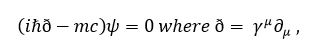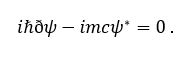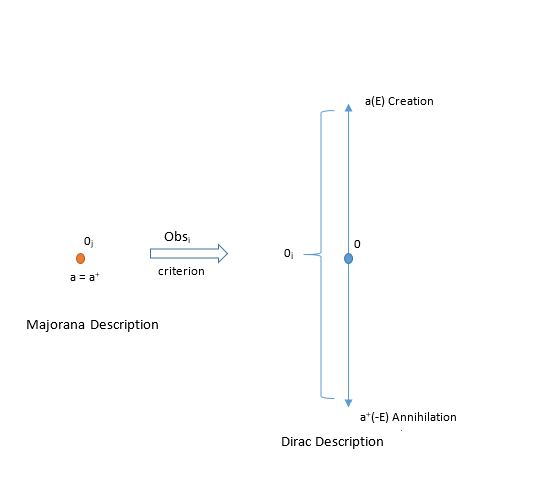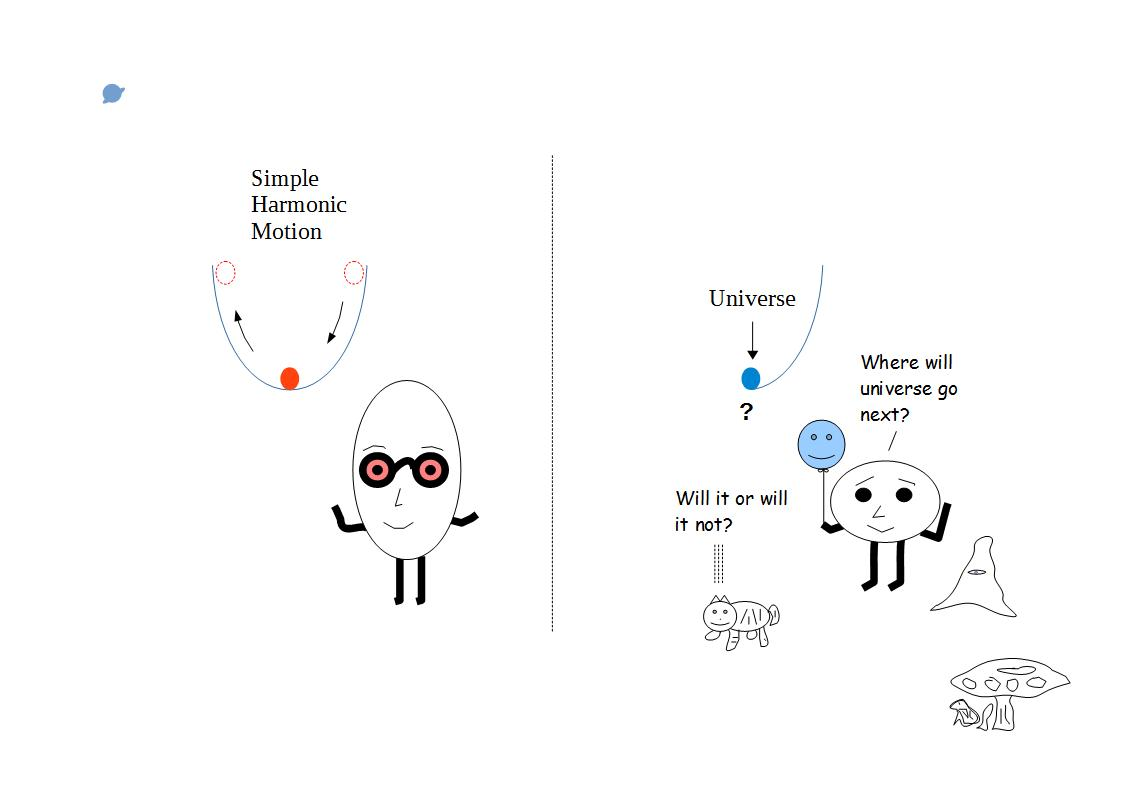# ijspace.org

 Majorana Fermions: j-space 15th May 2015       The following are two very well-known relativistic wave-equations representing essentially the same measurement Ψ,The first equation is Dirac equation which predicts the negative-energy states and the second equation is Majorana equation which does not require negative energy-states.  The particles satisfying Majorana equation are called Majorana particles or Majorana fermions.  One question which comes to mind right away is whether Dirac fermions and Majorana fermions truly represent the same measurement.  There is an ongoing debate whether most fundamental particles such as neutrinos are Dirac fermions or Majorana fermions.  (We have assumed that the complex algebra is a requirement for the relativistic description. Although it is an extremely powerful technique, we really do not have an intuitive explanation behind the use of the complex algebra in a physical description.)     The Majorana particles satisfy the following properties, a+ = a , Ψanti-particle = Ψ*particle  .     Please note that in Dirac description creation and annihilation operators a+ and a are not equal, but adjoint of each other. Unlike Dirac description, the wave-function Ψ  has to be neutrally charged in Majorana description and it does not couple with EM field.  In j-space description it means that Ψ representing Majorana particle is a state with information content which can not be measured in QED space by ObsM, an observer of finite capacity.  Both the Majorana particles and anti-particles are measured as neutral by ObsM .  However it does not mean that ObsM will not be affected by Majorana states.  The observer ObsM represents a physical entity which is always in a state of measurement.  ObsM  can be a human, a machine, a rock, or a galaxy.  Majorana states will instantaneously affect each of them alike.     Let us consider the condition a+ = a  and its significance in energy space.  In the following diagram the Majorana description in j-space is shown on the left.  If we apply the Obsi criterion then 0j actually turns out to be finite.  It is represented by an interval (-E, +E) and leads to Dirac description.  Energy is an entropy dependent concept in the observer's measurement metric.  The Majorana description represents the limit of the observer's capacity to make measurements.The Axiom of  Foundation in j-space is defined by the relationship,      ¬∈ = ∈. It represents  the definition of quantum in the measurement space or in other words the limitations of the observer.  The Axiom of Foundation can be thought of as equivalent to the conditions for Majorana fermions.  Axiom of Foundation represents the measurement limit of the information state for ObsM, at which VT Symmetry is invoked to develop Dirac description.  So by the looks of things neutrinos are likely to be Majorana particles rather than Dirac Fermions.     Majorana fermions probably is not a correct term as it assumes the existence of spin and the exclusion principle while enforcing the condition a+ = a.  The exclusion principle is an extremely powerful mechanism in j-space.  It implies that no two measurements are ever alike unless they assumed as such by a symmetry condition, most primitive being VTS which defines the origin.  Majorana condition can be satisfied only outside the j-space.  We will address entities satisfying the Majorana condition as Majorana states.  Majorana states should be regarded as precursors to Dirac Fermions.  Both Majorana states and Dirac fermions describe components of a mechanism by which an observer of a limited capacity would measure a source with infinite information.     Please note that the term "infinite source" here does not refer to the infinite life-time of the source although it is implicit in the definition.  The time scale exists only for an observer making measurements.  The infinite time scale represents an observer with entropy making measurements, for example ObsM.     We can also have states possibly with more information content than the states measured by an observer in q = 3  (QED space) as Majorana states.  Consequently such very high information states can not be completely measured (i.e. PE1 measurements are not possible) by the observer.  Therefore as far as we (ObsMs) are concerned they may not exist because we do not see them, but they actually do.      An important experiment reported recently (Observation of Majorana Fermions in Ferromagnetic Atomic Chains on a Superconductor, Stevan Nadj-Perge, Ilya K. Drozdov, Jian Li, Hua Chen, Sangjun Jeon, Jungpil Seo, Allan H. MacDonald, B. Andrei Bernevig, and Ali Yazdani, Science 346, 6209 (2014).), formed the mono-atomic Fe chain on a superconducting Pb substrate.  As the iron acquired the superconducting properties, the zero energy states also appeared at the edge of the mono-atomic Fe wire.  If we consider the Cooper pair they are equivalent to an observer in q = 3 state and its reference Virtual Twin, forming the observer pair required to define the origin 0j.  We note that the observer pair which defines the origin of the measurement metric will have the maximum capacity in the measurement space.  Therefore the observer pair represents a zero-entropy or a zero-loss conservative process within the measurement metric, similar to superconductivity.  However both the members of superconducting process are distinct and hence Majorana condition is not fulfilled.     Majorana condition is the threshold for the onset of VT Symmetry defining origin or the formation of the maximum capacity observers.  For example our friend Zörk who belongs to q = 2 space, will also follow Majorana condition, though the measurements made by Zörk related to Dirac fermions will have different information content.  Would it make sense if we suggested that an observer whose measurement metric has an inherent curvature, would represent a non-conservative process?  And that all the measurement metrics in j-space would always have some curvature per Obsi criterion?    As promised earlier, we shall start discussing Anharmonic Coordinates next.Information on www.ijspace.org is licensed under a Creative Commons Attribution 4.0 International License.